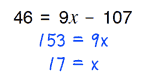# Home | Mathematics | Pre-Algebra

## Multiplication & Division

These equations are solved by combining like terms, followed by the same logic as two-step equations. Terms with the variable will be together on one side of the equation. All problems resolve to integers. You can adjust the difficulty of the problems by increasing the values size, using negative coefficients and flipping the sides of the equations.

## Multiplication & Division Options:

 Negative Coefficients:With NegativesAvoid Negatives Paper size: US Letter (8.5 x 11 in)   A4 (210 x 297 mm)